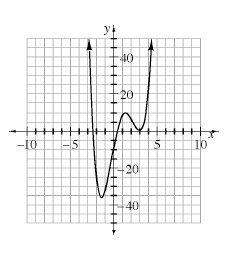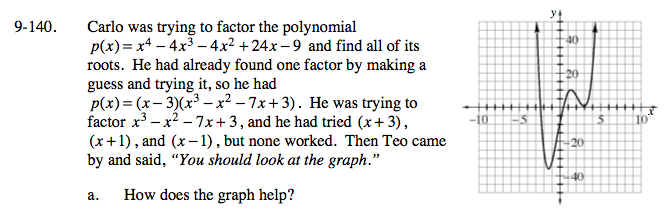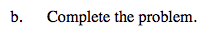Home > A2C > Chapter 9 > Lesson 9.3.2 > Problem9-140

9-140.
1. Carlo was trying to factor the polynomial p(x) = x4 − 4x3 − 4x2 + 24x − 9 and find all of its roots. He had already found one factor by making a guess and trying it, so he had p(x) = (x − 3)(x3x2 − 7x + 3). He was trying to factor x3x2 − 7x + 3, and he had tried (x + 3), (x + 1), and (x − 1), but none worked. Then Teo came by and said, “You should look at the graph.” Homework Help ✎1. How does the graph help?

2. Complete the problem.It shows us that 3 is a double root which means (x − 3)2 is a factor.Divide x3x2 − 7x + 3 by x − 3.

Use a multiplication rectangle to set it up.

Now use the quadratic formula to solve for x.

$x=3,-1\pm\sqrt{2}$